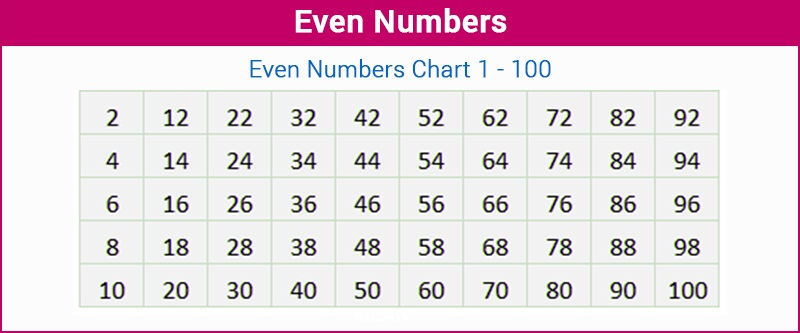# Java Program To Check Even Numbers | 4 Ways

Java program to check even numbers – In this article, we will discuss all the means to calculate even numbers in Java programming.

Suitable examples and sample programs have been added to the given article. The compiler has also been added so that you can execute the programs easily.

The means used in this piece are as follows:

Even numbers, as we all know, are any number which is completely divisible by two.As you can see in the image uploaded above, these are the even numbers that fall between 1 – 100.

Since the definition of even numbers say that there shouldn’t be a remainder, thus, 0 is also an even number.

Hence, the multiple methods to calculate even numbers in Java programming are as follows:

1.

Output:
2.
Output:
3.
Output:
4.
Output:
x

## Plus Star Pattern Java Program | Patterns

Java program to print plus star pattern program – We have written the below print/draw ...